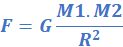USE PROMO CODE "DEMO100" TO AVAIL FREE DEMO CLASSGravitation

It is a natural phenomenon by which all things with mass and energy including planets, stars, moons, galaxies etc. are attracted to one another.

We all feel the gravitation of earth as we live on it. It pulls us with a force equal to our weight. Weight of all the physical objects and the atmosphere etc. on earth is because of the gravity. Tides in the ocean are caused because of the gravity of the moon.

Newton’s theory of gravitation

Through time many scientists have theorised about gravity or gravitation and English mathematician Sir Isaac Newton is considered one of the greatest contributor. He in 1687 published Principia which hypothesizes the inverse square law of Universal gravitation. The equation is the following:Where           F = gravitational force between two objects

M1, M2 = mass of the two objects

R = distance between the two objects

## Join As Tutor Or Student

Join our community that have more than 10,000 subscribers and learn new things everyday